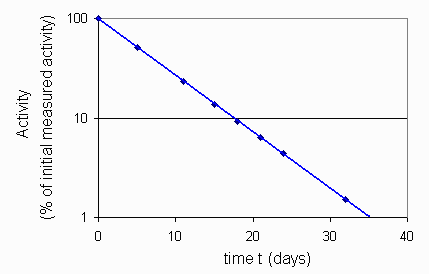# Nuclear Medicine: Fitting a non-linear Equation to Data by Linearizing

This example is adapted from an article on "Monitoring Radioactive Xenon Gas in Room Air Using Activated Charcoal", Journal of Nuclear Medicine Technology, March 1990.

The purpose of the study was to investigate the use of activated charcoal as a means of monitoring radiation exposure to the staff while performing Xenon-133 studies. Xenon-133 is widely used for brain and blood flow measurements. It is exhaled by patients during the study. The expired Xenon -133 must be contained by a face mask or vented to the atmosphere without exposing the technologist performing the evaluation.

Part of the study involved setting up a reference standard from which efficiency of the adsorption onto activated charcoal could be judged.

111 MBq of Xenon-133 was heat sealed into a tube and the exact amount of radioactivity was measured in a dose calibrator periodically for 31 days. The results are as shown:

### Xenon-133 Standard Decay in Dose Calibrator

time t
(days)
Activity A
(% of Initial Measured Activity)
0 100
5 51.7
11 23.5
15 13.8
18 9.2
21 6.3
24 4.4
32 1.5

Here is a graph of the above data. Notice that the vertical axis has been logged.## Problem:

Find an equation containing no logarithms to represent this observed data. Be sure and identify any transformations you may have applied to the independent and dependent variables.

## Solution:

### Technical Notes:

1. Nuclear Medicine technologists would know that radioactive decay has an exponential relationship, typically given by:

A = A0e-λ t  (equation 1)

where λ and Ao are constants. Our job in this case is to solve for Ao and λ.
2. If you log or ln (natural logarithm) both sides of an exponential equation such as that shown in equation (1), you get a linear equation.

Let x represent the time t (in days) and y represent the natural logarithm of the % of initial measured activity, ln(A). These are the transformations we will apply to the data to solve for the two constants.

Then using the formula for a straight line, y = bx + a and applying the transformations, we get a linear equation, ln(A) = bt + a.

Now to solve for b and a,

• you can get an approximate "eyeball" answer by picking any two points off the graph of the line shown. For example, you might pick values (21, 6.3) and (5, 51.7) which could then be substituted for t and A into the equation for a straight line giving two equations in two unknowns to solve.

ln(6.3) = 21b + a

ln 51.7 = 5b + a

Subtracting the second equation from the first and using our calculator on the logs we get:

-2.104908 = 16 b

b= -0.131556759

Substituting into the first equation and solving for a gives a = 4.603241578

Thus the equation becomes

ln(A) = -0.13156 t + 4.60324

you can also use the preferred method which is to apply the method of Least Squares to the data. This is a built in function on most calculators. In this case,

b = -0.130992968, a = 4.598029229

Now we still have the problem of expressing the equation so that it contains no logarithms.

ln A= -0.13156 t + 4.60324

Antilog, recalling that:

ax + y = ax · ay